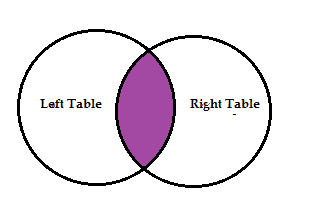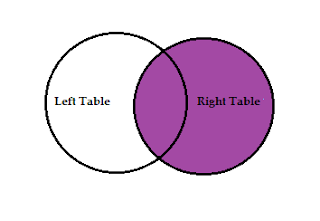# SQL- Join

## What is SQL Join?

SQL join is used to retrieve data from two or more tables in a database.SQL Join

#### Syntax:-

```SELECT column_names
FROM table_name1 JOIN table_name2
WHERE condition;```

#### Example: In the figure below we have two tables Customer and Order.

Now, let us join the two tables with the help of SQL Query and see what the output is.

Query :
```
FROM customers JOIN orders
WHERE customers.ID = orders.customers_Id;```
Output :

## Types of SQL Joins

• Inner Join:- Returns the records by matching the values of both the given tables.

####• Left Join:- Returns all records in the Left table by matching the Right table.

• Right Join:- Returns all records in the Right table by matching the Left table.

• Full Join:-  Returns all records when there is a match in the left or right table.

## 1. Inner Join

Inner Join returns the records by matching the values of both tables given with join.
Join keyword and Inner Join are both similar.Inner Join

#### Syntax:-

```SELECT   table1.column_name,  table.2column_name
FROM table_name1  INNER JOIN table_name2
ON   table1.common_field  = table1.common_field;```

#### Example: In the figure below we have two tables Customer and Order.

Now, let see join these two tables using the INNER JOIN as follows :

Query :

`SELECT ID, Name, AddressFROM customers  INNER JOIN ordersON customers.ID = orders.customers_Id;`
Output :

## 2. Left Join

In LEFT JOIN if all records of the LEFT table are returned by matching RIGHT table.
If the RIGHT table is not matched the 'NULL' (no value) return.Left Join

#### Syntax:-

```SELECT   table1.column_name,  table.2column_name
FROM table_name1  LEFT JOIN table_name2
ON   table1.common_field  = table1.common_field;```
Examplefigure below, we have, two tables customers and orders.

Now, let see join these two tables using the LEFT JOIN as follows.

Query
`SELECT Id, Name, AddressFROM customers LEFT JOIN ordersON customers.Id = orders.customers_Id;`
Output:

## 3. Right Join

In RIGHT JOIN if all records of the RIGHT table are returned by matching LEFT table.
If the LEFT table is not matched the 'NULL' (no value) return.Right Join
Syntax:-
```SELECT   table1.column_name,  table.2column_name
FROM table_name1  RIGHT JOIN table_name2
ON   table1.common_field  = table1.common_field;```
Example figure below, we have, two tables customers and orders.

Now, let see join these two tables using the RIGHT JOIN as follows.

Query:
`SELECT Id, Name, AddressFROM customers RIGHT JOIN ordersON customers.Id = orders.customers_Id;`

## 4. Full Join

FULL JOIN, Whether or not there are matches in the LEFT table and RIGHT table in FULL JOIN, it returns all records of both tables. If the match does not happen then 'NULL' (no value) is returned.
FULL OUTER JOIN and FULL JOIN is the same.
FULL JOIN has the possibility of returning a very large result-set.Full JOIN

Syntax:
```SELECT   table1.column_name,  table.2column_name
FROM table_name1  FULL JOIN table_name2
ON   table1.common_field  = table1.common_field;```

## 5. Self Join

Self Join This is a regular join, Self Join is used to join a table to itself. There is no keyword for this join.

#### Syntax:

```SELECT   a.column_name b.column_name
FROM table1a, table1b
WHERE   a.common_field  = b.common_field;```
Example: Figure in below we have customers table. Let's see how to work self Join in the database.

Now, let see join this table using SELF JOIN as follows

Query :
```SELECT   a.Id b.Name, a.Address
FROM customers a, customers b